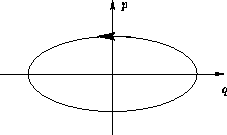Up: Microscopic and Macroscopic States. Previous: Time Evolution and Ergodicity

# Quiz

If you do not know some of the terms below, just leave blanks. The answers are not graded!

1.
Calculate entropy for the states M=-3, M=-1, M=1 and M=3 for three spin Ising model.
2.
Calculate entropy for the states M2=1 and M2=9 for the three spin Ising model. Which state is more probable at high temperatures? Why?
3.
Which of the following quantities are extensive and which are intensive:
(a)
Chemical potential
(b)
Osmotic pressure
(c)
Free energy
(d)
Concentration

4.
Gibbs postulate means that:
(a)
All ensembles are equivalent
(b)
All points in the phase space are equivalent
(c)
All points in the microcanonical ensemble are equivalent
(d)
The energy is conserved
5.
There is a mistake in the sketch of phase space of harmonic oscillator in the lecture notes:Find it.

© 1997 Boris Veytsman and Michael Kotelyanskii
Wed Sep 3 22:59:36 EDT 1997